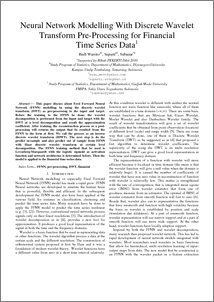# Neural Network Modelling With Discrete Wavelet Transform Pre-Processing for Financial Time Series Data

Warsito, Budi and Suparti, Suparti and Subanar, Subanar (2010) Neural Network Modelling With Discrete Wavelet Transform Pre-Processing for Financial Time Series Data. Departemen Statistika .Preview
PDF
118Kb

## Abstract

This paper discuss about Feed Forward Neural Network (FFNN) modelling by using the discrete wavelet transform (DWT) as pre-processing to the input and target. Before the training to the FFNN be done, the wavelet decomposition is performed from the input and target with the DWT at a level decomposition and result the approximation coefficient. After training, the reconstruction process as a postprocessing will returns the output that be resulted from the FFNN to the term at first. We call the process as an inverse discrete wavelet transform (IDWT). The next step is do the predict in-sample and also predict out of sample from FFNN with Haar discrete wavelet transform at certain level decomposition. The FFNN training method that be used is Levenberg-Marquardt with the logistic sigmoid as activation function and network architectur is determined before. Then the model is applied to the financial time series data.

Item Type: Article H Social Sciences > HA Statistics Faculty of Science and Mathematics > Department of Statistics 80191 Mr Hasbi Yasin 26 Feb 2020 19:44 27 Feb 2020 21:07

Repository Staff Only: item control page